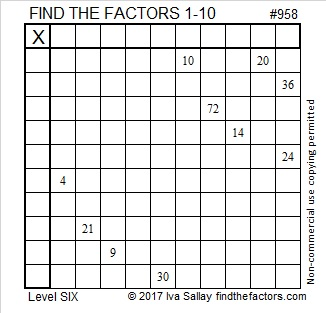# 958 and Level 6

This puzzle is a multiplication table. You don’t have to be fast to solve it, but you do have to think. There is only one solution. The ten clues given are sufficient to find the places to put the factors 1 to 10 in the first column and the top row. Good luck!Print the puzzles or type the solution in this excel file: 10-factors-951-958

958 is the sum of the 22 prime numbers from 5 to 89.

958 is also 141 in BASE 29 because 1(29²) + 4(29¹) + 1(29⁰) = 958

• 958 is a composite number.
• Prime factorization: 958 = 2 × 479
• The exponents in the prime factorization are 1 and 1. Adding one to each and multiplying we get (1 + 1)(1 + 1) = 2 × 2 = 4. Therefore 958 has exactly 4 factors.
• Factors of 958: 1, 2, 479, 958
• Factor pairs: 958 = 1 × 958 or 2 × 479
• 958 has no square factors that allow its square root to be simplified. √958 ≈ 30.951575This site uses Akismet to reduce spam. Learn how your comment data is processed.